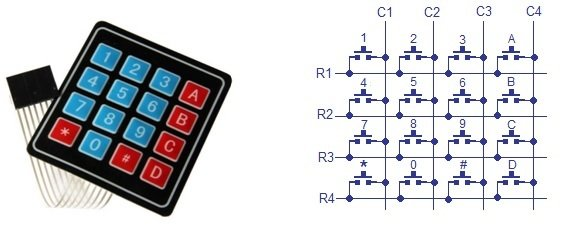# KEYPAD INTERFACING 8051 MICROCONTROLLER with code

In a keypad, push button switches are arranged in rows and columns. For a 4×4 keypad 16 switches are used and to connect to microcontroller we need 16 inputs pins. But the arrangement is changed by connecting switches in a special way. Now we need only 8 pins of microcontroller to connect keypad to it.The status of each key/switch is determined by Scanning the rows or columns. The column pins (Col 1–Col4) are connected to the microcontroller as the inputs pinsand the rows pins (Row 1–Row 4) are connected to the output pins of the microcontroller. Normally, all the column pins are pulled high by internal or external pull up resistors. Now we can read the status of each switch through scanning.

Scanning is done in a different way. Columns pins are used as input pins, and rows pins are used as output. If a low logic is given to all the Rows and high logic is given to each Column.

For finding Column number:

• When a switch/key is pressed, the corresponding row and column will get short.
• Output of the corresponding column goes to go low.
• Since we have made all the rows zero so this gives the column number of the pressed key.

For Finding Row number:

• After the detection of column number, the controller set’s all the rows to high.
• Each row is one by one set to zero by the microcontroller and the earlier detected column is checked and obviously it becomes zero.
• The row due to which the column gets zero is the row number of the pressed key.

For the interfacing of keypad with the microcontroller, it is good to connect LCD also, so that we can observe specific changes if the keypad is pressed. Keypad 4×3 (having 4 rows and 3 columns) is connected to Port 3 of 8051 microcontroller to scan input. LCD is connected to Port 2 of the microcontroller for displaying output. Port1 pin1and pin2 of microcontroller is connected to RS and EN pins of LCD respectively. LED is connected to port 1 pin 3.

### PROGRAMMING STEPS of  keypad interfacing 8051 microcontroller

1. Firstly circuit will be initailized by declaring ports as inputs or outputs. P1, P2 are used as output ports. P3 lower bits are used as outputs while upper bits as inputs.
2. Then LCD is initialized by specific commands.
3. All columns are given high logic and all rows are given low logic.

col1=1;

col2=1;

col3=1;

row1=row2=row3=row4=0;

1. Externally, when any keypad button is pressed, specific column output goes low. Check each column for it.

if(col1==0) check_col1();

else if(col2==0) check_col2();

else if(col3==0) check_col3();

1. For that specific column, check each row one by one. Give low logic to single row and high logic to other rows, repeat this process for other rows and row is found.

For Example:

• In pressing of 1, Col 1 goes low.
• For Col 1 check the row1. (give low logic to row1 and high to others)

row1=row2=row3=row4=1;
row1=0;

if(col1==0){writecmd(0x01); led = 1; writedata(‘1’); delay(5000000); led = 0;}

### WORKING of keypad interfacing 8051 microcontroller

In the start, LCD will display “WELCOME” and then display “Enter No.”. In above screenshot, I pressed 2. It is then written on LCD. When button is pressed, Led will get on and then then off after some delay. The number will appear on the screen as long as other number is not pressed. This display arrangement can be changed by arranging the code in different way.

### CODE of keypad interfacing 8051 microcontroller

```#include<reg51.h>

voidlcd_init(void);

voidwritecmd(int);

voidwritedata(char);

void delay(int);

void check_col1();

void check_col2();

void check_col3();

sbit RS = P1^0;

sbit E  = P1^1;

sbit led = P1^2;

sbit row1=P3^0;

sbit row2=P3^1;

sbit row3=P3^2;

sbit row4=P3^3;

sbit col1=P3^4;

sbit col2=P3^5;

sbit col3=P3^6;
inti=0;
void main()

{

P0 = 0x00;   //not used

P1 = 0x00;   //output port for setting RS and EN

P2 = 0x00;   //used as data output port   for LCD

P3 = 0xf0;   //lower bits as outputs, upper bits as inputs

lcd_init();

writedata('W');

delay(5000000);

writedata('e');

delay(5000000);

writedata('l');

delay(5000000);

writedata('c');

delay(5000000);

writedata('o');

delay(5000000);

writedata('m');

delay(5000000);

writedata('e');

delay(5000000);
writecmd(0x01);                               //clear display
writedata('E');

writedata('N');

writedata('T');

writedata('E');

writedata('R');

writedata(' ');

writedata('N');

writedata('o');

writedata('.');

delay(5000000);

do

{

col1=1;

col2=1;

col3=1;

row1=row2=row3=row4=0;

if(col1==0) check_col1();

else if(col2==0) check_col2();

else if(col3==0) check_col3();

}while(1);

}

voidlcd_init(void)

{

writecmd(0x38);    //for 8 bit mode

writecmd(0x0C);    //display on, cursor off

writecmd(0x01);    //clear display

writecmd(0x80);    //force cursor to beginning of 1st line

}
void writedata(char t)     //data function

{

RS = 1;

P2 = t;                                //Data transfer

E  = 1;

delay(150);

E  = 0;

delay(150);

}

voidwritecmd(int z)        //command function

{

RS = 0;

P2 = z;                                //Data transfer

E  = 1;

delay(150);

E  = 0;

delay(150);

}

void delay(int a)               //Delay function

{

inti;

for(i=0;i<a;i++);

}

void check_col1() //Function for checking column one

{

row1=row2=row3=row4=1;

row1=0;

if(col1==0){writecmd(0x01); led = 1; writedata('1'); delay(5000000); led = 0;}

row1=1;

row2=0;

if(col1==0){writecmd(0x01); led = 1; writedata('4'); delay(5000000); led = 0;}

row2=1;

row3=0;

if(col1==0){writecmd(0x01); led = 1; writedata('7'); delay(5000000); led = 0;}

row3=1;

row4=0;

if(col1==0){writecmd(0x01);led = 1; writedata('*'); delay(5000000); led = 0;}

row4=1;

}

void check_col2() //Function for checking column two

{

row1=row2=row3=row4=1;

row1=0;

if(col2==0){writecmd(0x01);led = 1; writedata('2'); delay(5000000); led = 0;}

row1=1;

row2=0;

if(col2==0){writecmd(0x01);led = 1; writedata('5'); delay(5000000); led = 0;}

row2=1;

row3=0;

if(col2==0){writecmd(0x01);led = 1; writedata('8'); delay(5000000); led = 0;}

row3=1;

row4=0;

if(col2==0){writecmd(0x01);led = 1; writedata('0'); delay(5000000); led = 0;}

row4=1;

}

void check_col3() //Function for checking column three

{

row1=row2=row3=row4=1;

row1=0;

if(col3==0){writecmd(0x01); led = 1; writedata('3'); delay(5000000); led = 0;}

row1=1;

row2=0;

if(col3==0){writecmd(0x01); led = 1; writedata('6'); delay(5000000); led = 0;}

row2=1;

row3=0;

if(col3==0){writecmd(0x01); led = 1; writedata('9'); delay(5000000); led = 0;}

row3=1;

row4=0;

if(col3==0){writecmd(0x01); led = 1; writedata('#'); delay(5000000); led = 0;}

row4=1;

}```

### 2 thoughts on “KEYPAD INTERFACING 8051 MICROCONTROLLER with code”

1. I’m getting an error in Proteus simulation like no model specified for RV1